• Call Now

1800-102-2727•

# Coefficient of Viscosity- Introduction of Viscosity, Definition, Unit of Coefficient of Viscosity, Factor Affecting Viscosity and Applications

Did you observe that when you try to pour honey on a plate it does not flow normally and appears to be thick and sticky. On the other hand, if you throw water on the floor, it will flow to a larger distance. What do you think may be the reason for this difference in their nature? Let me explain the reason for it. This difference in the flow of honey and water is due to the attractive forces present between the layers. A higher attraction force between the layers does not allow the substance to flow and is known as a viscous liquid. French scientist Jean-Leonard Marie Poiseuille led the development of the measurement of viscosity because of his curiosity to know about the circulation of blood in the human body.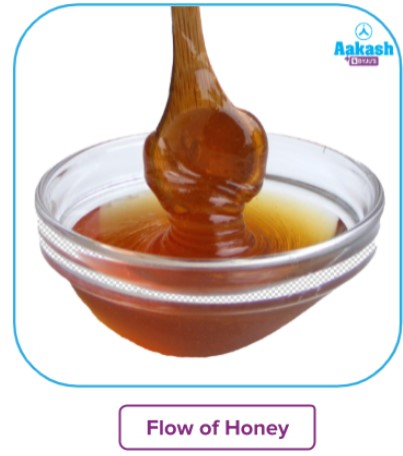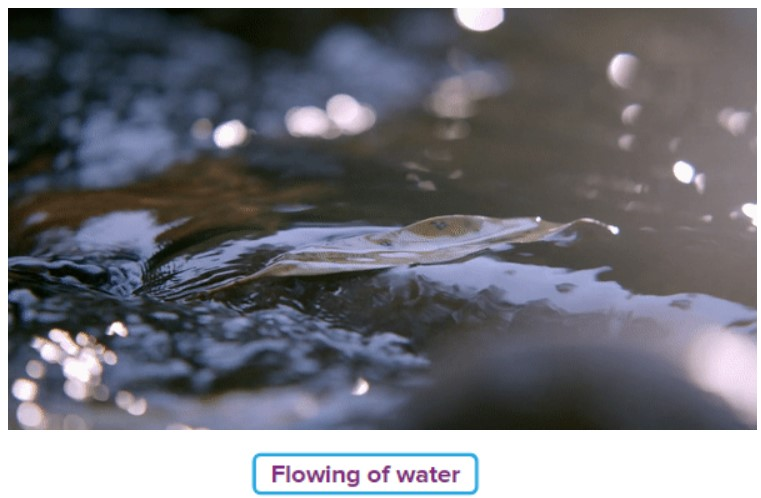• Introduction to Viscosity
• Definition and Formula of Coefficient of Viscosity
• Unit of Coefficient of Viscosity
• Factors Affecting Viscosity
• Application of Viscosity
• Practice Problems
• Frequently Asked Questions - FAQs

## Introduction to Viscosity

Viscosity is the degree to which a fluid can resist its flow under an applied force. It is measured by dividing the tangential frictional force acting per unit area by the velocity gradient under the condition of streamlined flow.

In simple words, it explains the fluid’s flow resistance. Viscosity is a significant rheological measurement closely related to the resistance to flow. Liquids like water, alcohol and petrol flow freely and faster than honey, glycerin and oil. This is because water, alcohol and petrol are less viscous than honey, glycerin and oil.

## Definition and Formula of Coefficient of Viscosity

The viscosity of a fluid is calculated in terms of its coefficient of viscosity. It is constant for a liquid and is dependent on the liquid’s nature, temperature, pressure and distance between the layers of the liquid. Poiseuille’s method is used to measure the coefficient of viscosity.

The main reason behind the calculation of viscosity is that the viscosity data on a material gives manufacturers a chance to predict how the material will react and behave when users or other components interact with it. General formula for measuring viscosity is given as follows: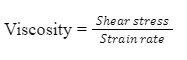Shear stress: It is defined as a force which causes the deformation in the object, parallel to a plane of t object.

Strain rate: It is defined as the difference in velocity between two layers of a fluid divided by the distance between the layers.The formulation of the coefficient of viscosity is done by assuming the force of friction between the two layers of the liquid having an area of square centimetres and also is separated by its distance. The relation is given as follows: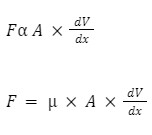Here,

F is the tangential force present between two layers of the liquid

A is the area of each layer of the liquid

µ is the coefficient of viscosity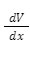is the velocity gradient

## Unit of Coefficient of Viscosity

As we know the tangential force between two layers of the liquid and coefficient of viscosity is related as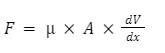Rearranging the above equation we get,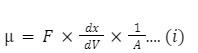Let,

The unit of the tangential force present between two layers of the liquid be Newton (N)

Unit of the area of each layer of the liquid be (m2)

The unit of the velocity gradientwill be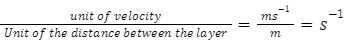Substituting the units of the known variable in the equation (i) we get,

Unit of coefficient of viscosity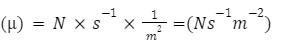## Factors Affecting Viscosity

There are different factors which affect the viscosity of liquid which includes:

• Nature of liquid: More the attraction force between the constituent layers of the liquid more will be the viscosity.
• Temperature: The viscosity of the liquid represent the attraction force present between the layers of the liquid. The more the attraction force between the layers, the more will be the viscosity. When the temperature of the substances is increased it helps in overcoming the attraction force between the different layers of the liquid. Therefore viscosity of the liquid decreases.
• Distance between the layers of the liquid: More the distance between the layers of the liquid, the lesser will be the attraction forces between the particles of the liquid and the lesser will be viscosity.
• Pressure: When the pressure increases the constituent layer comes closer so, more force of attraction and therefore generally it results in an increase in the viscosity of the liquid.

## Application of Viscosity

There is a lot of uses, especially in the industries that require the knowledge of viscosity for certain substances.

• Syrups developed by pharmaceutical companies check the viscosity of the syrups.
• Viscosity plays a huge role in developing paints and adhesives.
• Blood circulation depends on the viscosity of the fluids.
• The lubricant used for the machine part should have high viscosity.

## Practice Problems

Q 1. Which of the following options is correct with respect to the viscosity of blood and water?

a. The viscosity of blood is more than water
b. Water is more viscous than blood
c. Viscosity of blood and water cannot be compared
d. The viscosity of blood and water is equal

Viscosity is the measure of internal resistance present between the layers in the liquid and due to this friction it resists the flow of the liquid. In the case of blood and water blood has more viscosity than water and appears to be thicker than water.

Q 2. What is the C.G.S unit of coefficient of viscosity?

a. Candela
b. Poise
c. No unit
d. Newton

Candela is the S.I unit of luminous intensity, newton represents the S.I unit of force but poise is the C.G.S unit of viscosity given after the name of french scientist Jean-Leonard Marie Poiseuille.

As we know the tangential force between two layers of the liquid and coefficient of viscosity is related as:Rearranging the above equation we get,Let,

The unit of the tangential force present between two layers of the liquid be Newton(N)

Unit of the area of each layer of the liquid be (m2

The unit of the velocity gradientwill beSubstituting the units of the known variable in the equation (i) we get,

Unit of viscosityQ 3. A liquid having a velocity gradient 2.5 s-1 is filled in a container having a cross-sectional area 10 m2. Calculate the coefficient of viscosity of the liquid if the drag force between two adjacent layers is 1.5 N.

a.  0.5 Nsm-2
b. 1 Nsm-2
c. 0.375 Nsm-2
d. 1.3 Nsm-2

According to the given data,

Tangential force present between two layers of the liquid (F) = 1.5 N

Cross-sectional area between the layer of the liquid (A) = 10m2

Velocity gradient of the liquid () = 2.5 s-1

We know that coefficient of viscosity can be calculated using the equation which is represented as,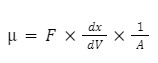Putting the value of the variables in the above equation, we get;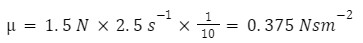Q 4. Select the correct option with respect to the viscosity of the liquid.

a. The viscosity of liquid increases with an increase in temperature.
b. The viscosity of the liquid does not depend on the nature of the liquid
c. The viscosity of liquid increases with an increase in pressure.
d.  Both A and B are correct.

There are different factors which affect the viscosity of liquid which includes:

• Nature of liquid: More the attraction force between the constituent layers of the liquid, the more will be the viscosity.
• Temperature: The viscosity of the liquid represent the attraction force present between the layers of the liquid. The more the attraction force between the layers, the more will be the viscosity. When the temperature of the substances is increased it helps in overcoming the attraction force between the different layers of the liquid. Therefore viscosity of the liquid decreases.
• Distance between the layers of the liquid: More the distance between the layers of the liquid, the lesser will be the attraction forces between the particles of the liquid and the lesser will be viscosity.
• Pressure: When the pressure increases the constituent layer comes closer so, more force of attraction and therefore generally it results in an increase in the viscosity of the liquid.

## Frequently Asked Questions - FAQS

Q 1 . What is the difference between viscosity and density?
Answer: Friction between the layers of the liquid that resists the flow of the liquid is known as the viscosity of the liquid. Whereas, the density of the substances is defined as the ratio of the mass of the substances per unit volume.

Q 2. What is the cause of viscosity in liquid?
Answer: The viscosity of a fluid is basically resistance to flow. As there are attraction forces present between the liquid particles which hold different layers present in the liquid and do not allow it to flow easily.

Q 3. What are the different types of viscosity of the liquid?
Answer: The viscosity of a fluid is a measurement of its ability to flow. Kinematic and dynamic viscosity are the two types of viscosity that are typically reported. The link between shear stress and shear rate in a fluid is known as dynamic viscosity. The link between viscous and inertial forces in a fluid is known as kinematic viscosity.

Q 4. Which among water, cooking oil and honey has the highest viscosity and least viscosity and why?
Answer: The viscosity of a fluid is basically resistance to flow. The higher the attraction force present between the liquid particles more will be the viscosity of the liquid. Viscosity is measured in terms of the coefficient of viscosity value. From the given list of substances, honey will have the highest viscosity and water will have the lowest viscosity.

Related topics

 Van der Waals equation Gay-Lussac’s Law Kinetic theory of gases Dalton's Law of Partial Pressure Ideal Gas Equation Factor affecting states of matterTalk to our expert
Resend OTP Timer =
By submitting up, I agree to receive all the Whatsapp communication on my registered number and Aakash terms and conditions and privacy policy